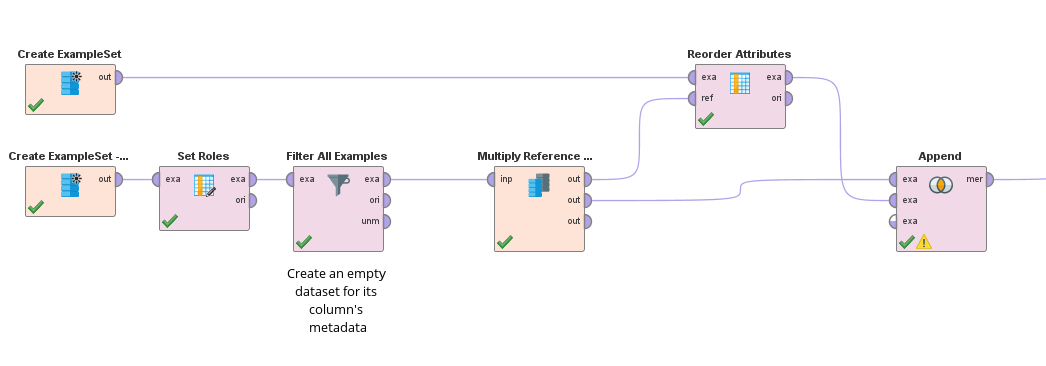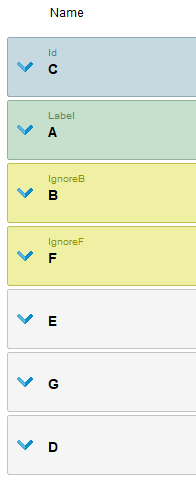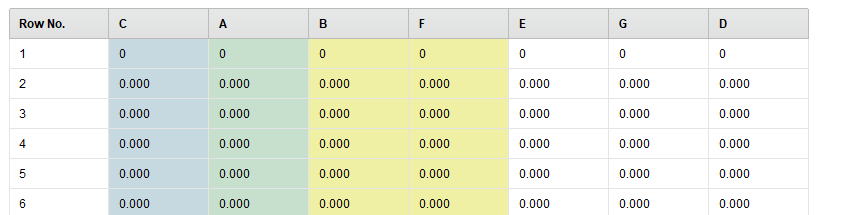# Get and set roles from a reference data set

Member Posts: 50Guru
I have a data set with various features that have been excluded by marking them with a role (they were not removed because they can be useful for reference even if they should be excluded from most operators). Now, I would like to apply the same roles to another data set that has the same columns (on which no roles have been set)

In Python, I was able to do this to achieve my objective:

``` def rm_main(data,refdata): <br>```
`&nbsp; data.rm_metadata = refdata.rm_metadata <br>`
`&nbsp; return data, refdata`

However, this can be slow for large data sets because the whole dataset is passed back and forth between Python and RapidMiner, which is not necessary in cases where the only thing I want to do is manipulate the columns metadata.

Is there a native way to do something similar with RapidMiner operators (or with an extension that adds such an operator)?

Otherwise, would the Groovy scripting operator be usable for this? I tried experimenting with it but could not find something that works.

Example (not functional, all attributes are seen to have a "null" role):

`ExampleSet inputData = input; <br>`
`ExampleSet referenceData = input; <br>`
```ExampleSetMetaData inputMetaData = operator.getInputPorts().getPortByIndex(0).getMetaData(); ExampleSetMetaData referenceMetaData = operator.getInputPorts().getPortByIndex(1).getMetaData(); <br>```
`for (Attribute attribute: referenceData.getAttributes()) { <br>`
`&nbsp; AttributeMetaData referenceAttributeMetaData = referenceMetaData.getAttributeByName(attribute.getName())`
```&nbsp; String referenceRole = referenceAttributeMetaData.getRole() <br>```
`&nbsp; LogService.root.log(Level.INFO, "Role for " + attribute.getName() + ": " + referenceRole); <br>`
`}`

Tagged:

• Member Posts: 50Guru
edited April 2020
I thought of a solution using both the Filter and Append operators, which happens to do what I want even if it's not made explicit. It seems to be working fine. I'm still curious about the feasibility of using the scripting operator however.

- Filter removes all rows from the "reference dataset": a dataset where the columns have the roles I want to set
- First input of the Append operator is the "reference dataset", second input is the actual data, with the same columns but without any role set

The resulting dataset will use the metadata of the first input (with the roles), but will include all rows from the actual data.```
<?xml version="1.0" encoding="UTF-8"?><process version="9.6.000">
<context>
<input/>
<output/>
<macros/>
</context>
<operator activated="true" class="process" compatibility="9.6.000" expanded="true" name="Process">
<parameter key="logverbosity" value="init"/>
<parameter key="random_seed" value="2001"/>
<parameter key="send_mail" value="never"/>
<parameter key="process_duration_for_mail" value="30"/>
<parameter key="encoding" value="SYSTEM"/>
<process expanded="true">
<operator activated="true" class="utility:create_exampleset" compatibility="9.6.000" expanded="true" height="68" name="Create ExampleSet" width="90" x="179" y="85">
<parameter key="generator_type" value="numeric series"/>
<parameter key="number_of_examples" value="1000000"/>
<parameter key="use_stepsize" value="false"/>
<list key="function_descriptions"/>
<list key="numeric_series_configuration">
<parameter key="A" value="linear.0\.0.1\.0"/>
<parameter key="C" value="linear.0\.0.1\.0"/>
<parameter key="F" value="linear.0\.0.1\.0"/>
<parameter key="B" value="linear.0\.0.1\.0"/>
<parameter key="D" value="linear.0\.0.1\.0"/>
<parameter key="G" value="linear.0\.0.1\.0"/>
<parameter key="E" value="linear.0\.0.1\.0"/>
</list>
<list key="date_series_configuration"/>
<list key="date_series_configuration (interval)"/>
<parameter key="date_format" value="yyyy-MM-dd HH:mm:ss"/>
<parameter key="time_zone" value="SYSTEM"/>
<parameter key="column_separator" value=","/>
<parameter key="parse_all_as_nominal" value="false"/>
<parameter key="decimal_point_character" value="."/>
<parameter key="trim_attribute_names" value="true"/>
</operator>
<operator activated="true" class="utility:create_exampleset" compatibility="9.6.000" expanded="true" height="68" name="Create ExampleSet - Reference data with roles" width="90" x="179" y="187">
<parameter key="generator_type" value="numeric series"/>
<parameter key="number_of_examples" value="100"/>
<parameter key="use_stepsize" value="false"/>
<list key="function_descriptions"/>
<list key="numeric_series_configuration">
<parameter key="A" value="linear.0\.0.1\.0"/>
<parameter key="B" value="linear.0\.0.1\.0"/>
<parameter key="C" value="linear.0\.0.1\.0"/>
<parameter key="D" value="linear.0\.0.1\.0"/>
<parameter key="E" value="linear.0\.0.1\.0"/>
<parameter key="F" value="linear.0\.0.1\.0"/>
<parameter key="G" value="linear.0\.0.1\.0"/>
</list>
<list key="date_series_configuration"/>
<list key="date_series_configuration (interval)"/>
<parameter key="date_format" value="yyyy-MM-dd HH:mm:ss"/>
<parameter key="time_zone" value="SYSTEM"/>
<parameter key="column_separator" value=","/>
<parameter key="parse_all_as_nominal" value="false"/>
<parameter key="decimal_point_character" value="."/>
<parameter key="trim_attribute_names" value="true"/>
</operator>
<operator activated="true" class="set_role" compatibility="9.6.000" expanded="true" height="82" name="Set Roles" width="90" x="313" y="187">
<parameter key="attribute_name" value="D"/>
<parameter key="target_role" value="regular"/>
<parameter key="B" value="ignoreB"/>
<parameter key="F" value="ignoreF"/>
<parameter key="A" value="label"/>
<parameter key="C" value="id"/>
</list>
</operator>
<operator activated="true" class="filter_examples" compatibility="9.6.000" expanded="true" height="103" name="Filter All Examples" width="90" x="447" y="187">
<parameter key="parameter_expression" value="false"/>
<parameter key="condition_class" value="expression"/>
<parameter key="invert_filter" value="false"/>
<list key="filters_list"/>
<parameter key="filters_logic_and" value="true"/>
<description align="center" color="transparent" colored="false" width="126">Create an empty dataset for its column's metadata</description>
</operator>
<operator activated="true" class="multiply" compatibility="9.6.000" expanded="true" height="103" name="Multiply Reference Data" width="90" x="648" y="187"/>
<operator activated="true" class="order_attributes" compatibility="9.6.000" expanded="true" height="82" name="Reorder Attributes" width="90" x="849" y="85">
<parameter key="sort_mode" value="reference data"/>
<parameter key="attribute_ordering" value=""/>
<parameter key="use_regular_expressions" value="false"/>
<parameter key="handle_unmatched" value="append"/>
<parameter key="sort_direction" value="ascending"/>
</operator>
<operator activated="true" class="append" compatibility="9.6.000" expanded="true" height="103" name="Append" width="90" x="1050" y="187">
<parameter key="datamanagement" value="double_array"/>
<parameter key="data_management" value="auto"/>
<parameter key="merge_type" value="all"/>
</operator>
<connect from_op="Create ExampleSet" from_port="output" to_op="Reorder Attributes" to_port="example set input"/>
<connect from_op="Create ExampleSet - Reference data with roles" from_port="output" to_op="Set Roles" to_port="example set input"/>
<connect from_op="Set Roles" from_port="example set output" to_op="Filter All Examples" to_port="example set input"/>
<connect from_op="Filter All Examples" from_port="example set output" to_op="Multiply Reference Data" to_port="input"/>
<connect from_op="Multiply Reference Data" from_port="output 1" to_op="Reorder Attributes" to_port="reference_data"/>
<connect from_op="Multiply Reference Data" from_port="output 2" to_op="Append" to_port="example set 1"/>
<connect from_op="Reorder Attributes" from_port="example set output" to_op="Append" to_port="example set 2"/>
<connect from_op="Append" from_port="merged set" to_port="result 1"/>
<portSpacing port="source_input 1" spacing="0"/>
<portSpacing port="sink_result 1" spacing="0"/>
<portSpacing port="sink_result 2" spacing="0"/>
</process>
</operator>
</process>

```